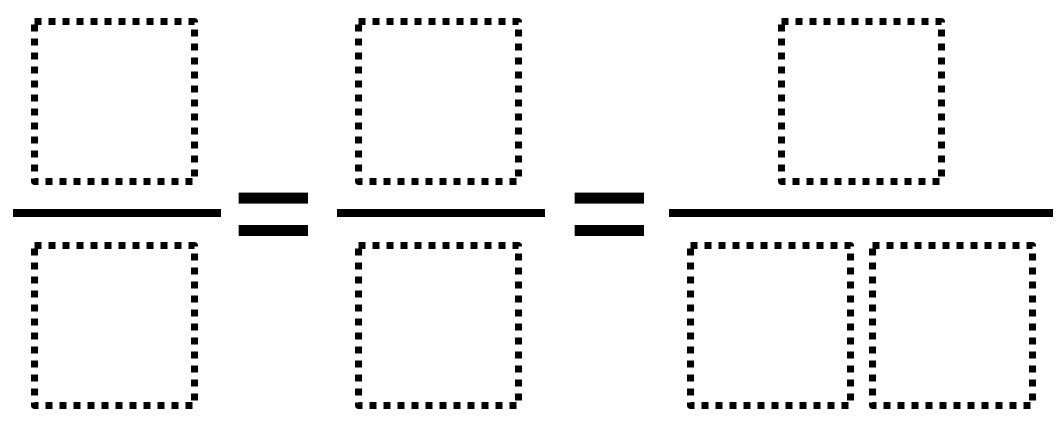Home > Grade 3 > Equivalent Fractions

# Equivalent Fractions

Directions: Use the digits 1 to 9, at most one time each, to make three equivalent fractions.### Hint

What must be true about all three fractions?

### Answer

There are many answers. Here are three possibilities:
6/8 = 3/4 = 9/12
2/4 = 3/6 = 9/18
2/8 = 1/4 = 9/36

Source: Owen Kaplinsky

## Baking Cookies

Directions: Daniel was making chocolate cookies. He had _ _ cookies in each row and …

### 4 comments

1.As far as my class could figure out the answers you present are the only three possibilities. We worked on creating organized lists to rule out all other possibilities.

When you start with the simplified version of the fraction:
-no numerators or denominators greater than 4 will work because when you multiply them by 2 they become a two digit number and we need two fractions with single digit denominators (i.e. 2/5 x 2= 4/10 two digit numerator – 2/6, x 2 = 4/12 – etc)
-The only possibilities for most simplified fraction to begin with are 1/2, 1/3, 1/4, 2/3, 3/4 – and 1/3 and 2/3 do not work
– 1/2: use 2/4, 3/6, 9/18
-1/3 doesn’t work
-1/4: 1/4, 2/8, 9/36
-2/3 – doesn’t work
-3/4: 3/4, 6/8, 9/12

2.I am curious why this task is tagged with Common Core 3.NF since Grade 3’s expectation in NF are limited to fractions with denominators of 2, 3, 4, 6, and 8. Would this task be better suited for Grade 4 where students work with denominators of 2, 3, 4, 5, 6, 8, 10, and 12?

3.That could work, but I think it is fine. Thanks for your opinion!